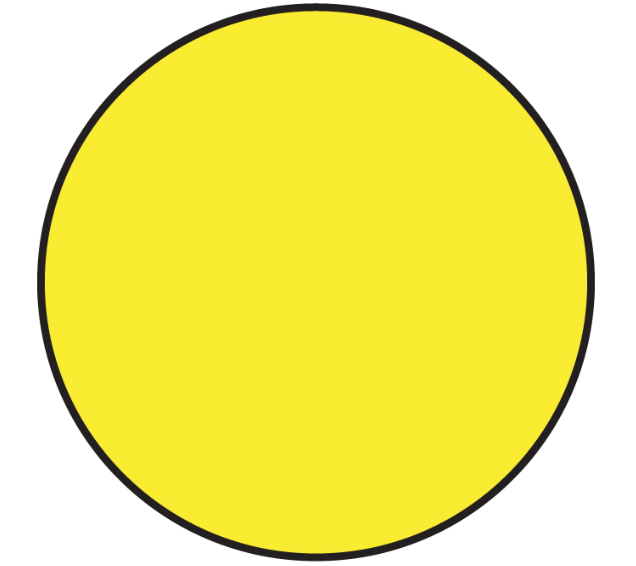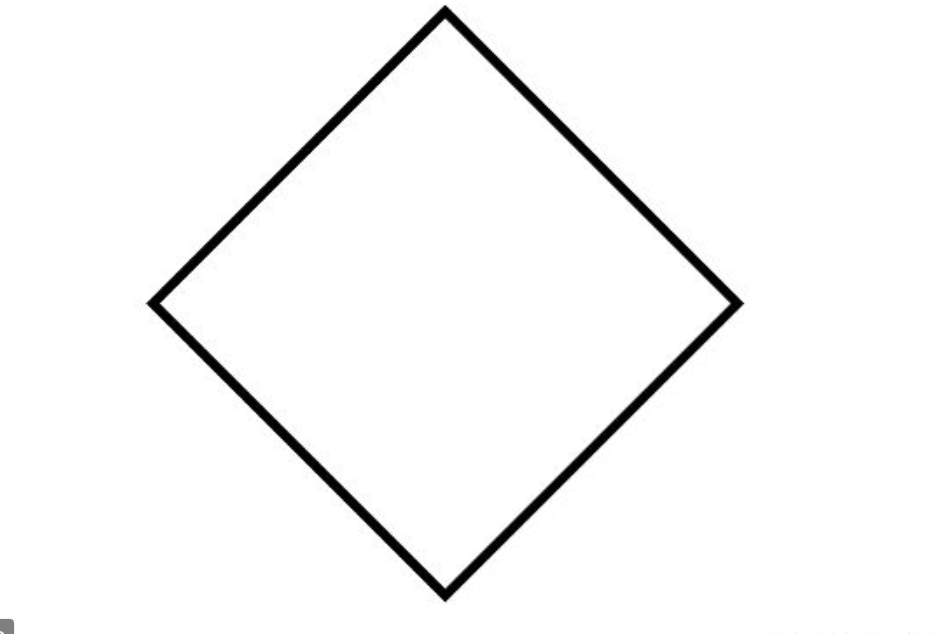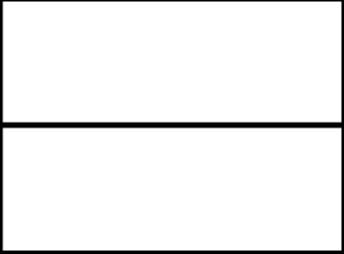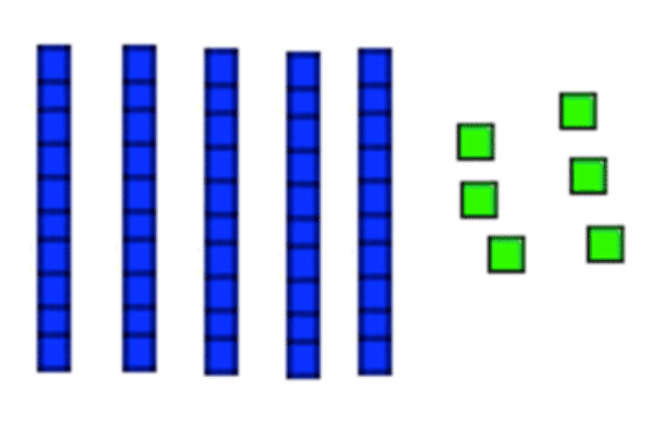Subtraction
Number Line
Shapes
Surprise!
100

8+7=

15

100

7-4=

3

100

What number is missing?

10,11,12,__,14

13

100

What shape is this?Circle

100

6+5=

11

200

9+9=

18

200

14-7=

7

200

What number is next?

21,22,23,24,__

25

200

What shape has three sides?

Triangle

200

15-6

9

300

10+7=

17

300

19-9

10

300

Which number is bigger?

43 or 44

44

300

What shape is this?Rhombus

300

What number is bigger?

56 or 59

59

400

3+3+6=

12

400

19-7=

12

400

What number is smaller?

24 or 32

24

400

This shape is cut in____?Halves

400

How much is this?56

500

15+7=

22

500

13-3-4

6

500

What number is missing?

99,100, __, 102, 103

101

500

This shape is cut in___?Fourths or quarters

500

12+3+4

19

Click to zoom